## RS Aggarwal Class 6 Solutions Chapter 13 Angles and Their Measurement Ex 13C

These Solutions are part of RS Aggarwal Solutions Class 6. Here we have given RS Aggarwal Solutions Class 6 Chapter 13 Angles and Their Measurement Ex 13C.

Other Exercises

Question 1.
Solution:
(i) Place the protractor in such a way that its centre is exactly at the vertex O of the given angle AOB and the base line lies along the arm OA. Read off the mark through which the arm OB passes, starting from 0° on the side A.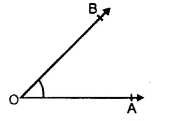We find that ∠AOB = 45°.
(ii) The given angle is ∠PQR. Place the protractor in such a way that its centre is exactly on the vertex Q of the given angle and the base line lies along the arm QR.Read off the mark through which the arm QP passes, starting from 0° on the side of R.We find that ∠PQR = 67°
(in) The given angle is ∠DEF. Place the protractor in such a way that its centre is exactly on the vertex E of the given angle and the base line lies along the arm ED. Read off the mark through which the arm EF passes, starting from 0° on the side of D.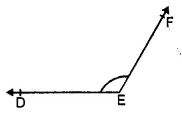We find that ∠DEF = 130°
(iv) The given angle is ∠LMN. Place the protractor in such a way that its centre is exactly on the vertex M of the given angle and the base line lies along the arm ML. Read off the mark through which the arm MN passes, starting from 0° on the side of L.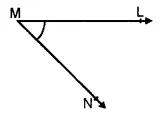We find that ∠LMN = 50°
(v) The given angle is ∠RST. Place the protractor in such a way that its centre is exactly on the vertex S of the given angle and the base line lies along the arm SR. Read off the mark through which the arm ST passes, starting from 0° on the side of R.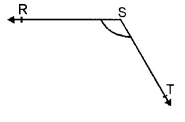We find that the ∠RST = 130°.
(vi) The given angle is ∠GHI. Place the protractor in such a way that its centre is exactly on the vertex H of the given angle and the base line lies along the arm HI. Read off the mark through which the arm HG passes, starting from 0° on the side of I.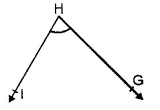We find that ∠GHI = 70°

Question 2.
Solution:
(i) Draw a ray OA. Place the protractor in such a way that its centre lies exactly at O and the base line lies along OA. Starting from 0° on the side of A, look for the 25° mark on the protractor. Mark a point B at this 25° mark. Remove the protractor and draw the ray OB. Then ∠AOB is the required angle.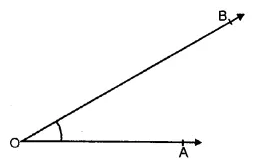(ii) Draw a ray OA. Place the protractor in such a way that its centre lies exactly at O and the base line lies along OA. Starting from 0° on the side of A, look for the 72° mark on the protractor. Mark a point B at this 72° mark. Remove the protractor and draw the ray OB. Then,
∠AOB is the required angle of measure 72°.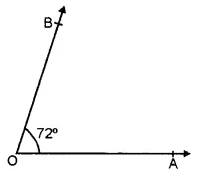(iii) Draw a ray OA. Place the protractor in such a way that its centre lies exactly at O and the base line lies along OA. Starting from 0° on the side of A, look for the 90° mark on the protractor. Mark a point B at this 90° mark. Remove the protractor and draw the ray OB. Then ∠AOB is the required angle whose measure is 90°.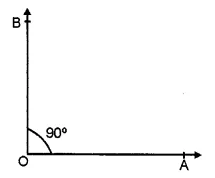(iv) Draw a ray OA. Place the protractor in such a way that its centre exactly lies at O and the base line lies along OA. Starting from 0° on the side of A, look for the 117° mark on the protractor. Mark a point B at this 117° mark. Remove the protractor and draw the ray OB. Then ∠AOB is the required angle whose measure is 117°.(v) Draw a ray OP. Place the protractor in such a way that its centre lies exactly at O and the base line lies along OP. Starting from 0° on the side of P, look for the 165° mark on the protractor. Mark a point Q at this 165° mark. Remove the protractor and draw the ray OQ. Then, ∠POQ is the required angle whose measure is 165°.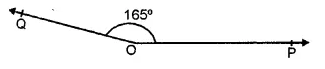(vi) Draw a ray OP. Place the protractor in such a way that its centre lies exactly at O and the base line lies along OP. Starting from 0° on the side of P, look for the 23° mark on the protractor. Mark a point Q at this 23° mark. Remove the protractor and draw the ray OQ. Then ∠POQ is the required angle whose measure is 23°.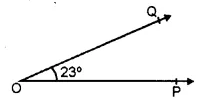(vii) Draw a ray OA. Place the protractor in such a way that its centre lies exactly at O and the base line lies along OA. Starting from 0° on the side of A,Took for the 180° mark on the protractor. Mark a point B on this 180° mark. Remove the protractor and draw the ray OB. Then ∠AOB is the required angle whose measure is 180°.(viii) Draw a ray Rs. Place the protractor in such away that its centre lies exactly at R and the base line lies along RS. Starting from 0° on the side of S, look for the 48° mark on the protractor. Mark a point T at this 48° mark. Remove the protractor and draw the ray RT. Then, ∠SRT is the required angle whose measure is 48°.Question 3.
Solution:
On measuring the given angle ABC with the help of a protractor, it is 50°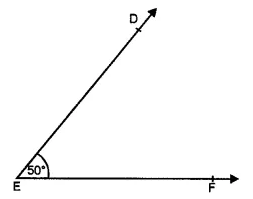Now, place the protractor on EF in such a way that its centre lies on E exactly and base with the line EF.
Now read off the mark through with the arm ED passes at 50°.
Join DE,
Then ∠DEF is equal to 50° i.e. equal to ∠ABC.

Question 4.
Solution:
Steps of construction :
(i) Draw a line segment AB = 6 cm.
(ii) Take a point C on AB such that AC = 4 cm.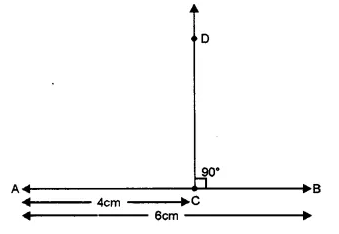(iii) Place protractor with its centre at C and base along CB.
(iv) Mark a point D against 90°.
(v) Remove the protractor and join DC. Then DC ⊥ AB. Ans.

Hope given RS Aggarwal Solutions Class 6 Chapter 13 Angles and Their Measurement Ex 13C are helpful to complete your math homework.

If you have any doubts, please comment below. Learn Insta try to provide online math tutoring for you.